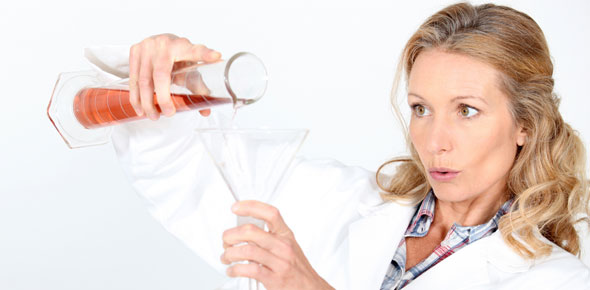# Types Of Reactions Quiz Questions

27 Questions | Total Attempts: 168SettingsReview for types of reactions and balancing equations

• 1.
A reaction that involves two ionic compounds in solution reacting to form two new compounds is a ? reaction.
• 2.
If a double replacement reaction is to occur, it must produce at least one of three different possibilities.  Which of the following is NOT one of the "driving forces" necessary for a double replacement reaction to take place?
• A.

Produces an insoluble solid (precipitate)

• B.

Produces a liquid

• C.

Produces a molecular compound such as water

• D.

Produces a gas

• 3.
A reaction in which one reactant breaks up into two or more products.
• 4.
A reaction in which a hydrocarbon reacts with oxygen producing water and carbon dioxide.
• 5.
A reaction in which only one product is formed.
• 6.
A reaction in which an element and a compound react and a new element and compound are formed.
• 7.
A term that means "dissolved in water"
• 8.
A whole number placed in front of a formula in a chemical equation in order to balance it.
• 9.
What kind of reaction will take place between sodium and a solution of magnesium chloride?
• A.

Single replacement

• B.

Double replacement

• C.

Combustion

• D.

Combination

• E.

Decomposition

• 10.
The balanced equation for the reaction between sodium and a solution of magnesium chloride is:(Make sure you check the chart in your book to see if this takes place!)
• A.

Na + MgCl --> Mg + NaCl

• B.

No Reaction

• C.

2 Na + MgCl2 --> Mg + 2 NaCl

• D.

Na + MgCl2 --> Mg + NaCl2

• 11.
What kind of reaction describes what happens when calcium is dropped into water?
• A.

Double replacement

• B.

Combustion

• C.

Combination

• D.

Decomposition

• E.

Single replacement

• 12.
The balanced equation for calcium and water is:
• A.

No reaction

• B.

2 Ca + 2 H2O --> H2 + Ca(OH)2

• C.

Ca + H2O --> 2 H + CaO

• D.

Ca + H2O --> H2 + CaO

• 13.
Which is the equation that would occur if silver was placed in a solution of potassium nitrate?
• A.

No reaction

• B.

Ag + KNO3 --> K + AgNO3

• C.

3 Ag + K3N --> 3 K + Ag3N

• D.

Ag + K2NO3 --> K2 + AgNO3

• 14.
What type of reaction would occur if hydrochloric acid was mixed with barium hydroxide?
• A.

Double replacement

• B.

Single replacement

• C.

Combustion

• D.

Combination

• E.

Decompostion

• 15.
What is the balanced equation for the reaction between hydrochloric acid and barium hydroxide?
• A.

HClO + BaOH --> BaClO + HOH

• B.

2 HCl + Ba(OH)2 --> 2 H2O + BaCl2

• C.

2 HCl + BaOH2 --> H2O + BaCl2

• D.

HCl + BaOH --> BaCl + HOH

• 16.
The reason that all chemical equations must be balanced is to obey the law of conservation of ?
• 17.
The law of conservation of mass states that:
• A.

Mass depends on the number and types of atoms present

• B.

Mass can increase, decrease or stay the same in a reaction

• C.

Mass cannot be created or destroyed

• D.

Mass is not the same as weight

• 18.
In incomplete combustion, a poisonous gas called ? is produced.
• A.

Carbon dioxide

• B.

Carbon monoxide

• C.

Carbon tetrachloride

• D.

Carbon

• 19.
In incomplete combustion, there is usually unburned ? that is produced.
• A.

Hydrogen

• B.

Carbon dioxide

• C.

Carbon

• D.

Water

• 20.
The complete combustion of C4H8 would be written as:
• A.

C4H8 --> CO2 + H2O

• B.

C4H8 + O2 --> CO2 + H2O

• C.

C4H8 + 6 O2 --> 4 CO2 + 4 H2O

• D.

C4H8 + O2 --> 4 H2 + 4 CO2

• 21.
The reaction between sodium and iodine would be ?
• A.

Double replacement

• B.

Single replacement

• C.

Combination

• D.

Complete combustion

• E.

Decompostion

• 22.
The equation for the reaction between sodium and iodine would be ?
• A.

Na + I --> NaI

• B.

Na + I2 --> NaI2

• C.

2 Na + I2 --> 2 NaI

• D.

Na + 2 I --> NaI2

• 23.
Aluminum bromide breaking up into its elements would be an example of ?
• A.

Decomposition

• B.

Combination

• C.

Single replacement

• D.

Double replacement

• E.

Combustion

• 24.
The balanced equation for aluminum bromide breaking up into its elements would be ?
• A.

AlBr --> Al + Br

• B.

AlBr3 --> Al + Br3

• C.

AlBr3 --> Al + 3 Br

• D.

2 AlBr3 --> 2 Al + 3 Br2

• 25.
Review: One mole of nitrogen gas would have a volume of ? liters at STP
Related Topics